# diskmarginplot

Visualize disk-based stability margins

Since R2020a

## Syntax

``diskmarginplot(L)``
``diskmarginplot(L1,...,LN)``
``diskmarginplot(L1,LineSpec1,...,LN,LineSpecN)``
``diskmarginplot(___,sigma)``
``diskmarginplot(___,w)``
``diskmarginplot(___,opts)``
``diskmarginplot(DGM)``
``diskmarginplot(DGM,'disk')``
``diskmarginplot(DGM,'nyquist')``
``diskmarginplot(alpha,sigma,___)``
``diskmarginplot(AX,___)``

## Description

### Margins as a Function of Frequency

example

````diskmarginplot(L)` plots the disk-based gain and phase margins for the SISO or MIMO negative feedback loop `feedback(L,eye(N))`, where `N` is the number of inputs and outputs in the open-loop response `L`.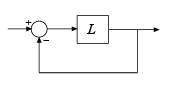For MIMO responses, `diskmarginplot` plots the multiloop disk margins. The disk-based gain margin at each frequency is ±GM, where GM is the value shown in the plot in dB. Similarly, the disk-based phase margin is ±PM degrees, where PM is the value shown on the plot. For details about disk-based gain and phase margins, see `diskmargin`.```

example

````diskmarginplot(L1,...,LN)` plots the disk-based gain and phase margins of multiple open-loop responses on the same plot.```

example

````diskmarginplot(L1,LineSpec1,...,LN,LineSpecN)` specifies a color, line style, and marker for each system in the plot.```
````diskmarginplot(___,sigma)` plots the disk-based gain and phase margins computed using the skew `sigma` to bias the gain variation toward gain increase (`sigma` > 0) or gain decrease (`sigma` < 0). If you have used `diskmargin` to obtain disk-based margins with some particular `sigma`, you can use this syntax to see the frequency dependence of the margins at that `sigma` value. For `sigma` ≠ 0, the plotted value is `GM = min(gmax,1/max(0,gmin))`. In other words, the plot shows the largest amount of gain change `[1/GM,GM]` that fits within the disk-based gain margin `[gmin,gmax]` of the system at the specified `sigma`. ```
````diskmarginplot(___,w)` plots the margins at the frequencies specified by `w`.If `w` is a cell array of the form `{wmin,wmax}`, then the plot shows the margins at frequencies ranging between `wmin` and `wmax`.If `w` is a vector of frequencies, then the plot shows the margins at each specified frequency.```

example

````diskmarginplot(___,opts)` uses specified options to customize plot elements such as labels, ticks, and grids. You can use this argument with any of the previous syntaxes.```

### Range of Gain and Phase Variations

example

````diskmarginplot(DGM)` plots the range of simultaneous gain and phase variations corresponding to a disk-based gain margin. The plot also shows the maximum gain-only and phase-only variations (this disk-based gain and phase margins). `DGM` is a vector of the form `[gmin,gmax]`. The gain margin `DGM` can also be a scalar, which is equivalent to specifying the symmetric gain variation `[1/DGM,DGM]`. To plot the ranges for multiple disk-based gain margins at once, use a two-column matrix of the form `[gmin1,gmax1;...;gminN,gmaxN]`. For more information about disk-based gain margins, see `diskmargin`. ```

example

````diskmarginplot(DGM,'disk')` plots the complex-valued disk of modeled gain and phase variations corresponding to the disk-based gain margin `DGM`. For details about how disk-margin analysis models gain and phase variations, see Stability Analysis Using Disk Margins. ```

example

````diskmarginplot(DGM,'nyquist')` plots the exclusion region in the Nyquist plane corresponding to the disk margin `DGM`. The requirement that the closed-loop system remain stable for gain or phase variations within the disk corresponding to `DGM` amounts to a requirement that the open-loop response remain outside a disk-shaped exclusion region in the Nyquist plane. For more information, see Stability Analysis Using Disk Margins.```

example

````diskmarginplot(alpha,sigma,___)` plots the range of gain and phase variations corresponding to the disk size `alpha` and skew `sigma`. If either `alpha` or `sigma` is a vector, then the plot includes the ranges for all specified values. If both `alpha` and `sigma` are vectors, then the plot includes the ranges for the pairs `alpha1,sigma1;...;alphaN,sigmaN`. To plot the modeled uncertainty disk corresponding to `alpha`,`sigma`, use this syntax with the `'disk'` flag. To plot the corresponding exclusion disk in the Nyquist plane, use this syntax with the `'nyquist'` flag.```

### Plot on Specified Axes

````diskmarginplot(AX,___)` draws a plot on the axes specified by an axes handle (`axes` or a `UIaxes` object). Use this argument to specify axes when creating apps in Develop Apps Using App Designer. You can use this argument with any of the previous syntaxes to draw any of the plots that `diskmarginplot` can generate.```

## Examples

collapse all

Plot the disk-based gain and phase margins of a system with open loop response `L` and closed-loop response `feedback(L,1)`.

```L = tf(25,[1 10 10 10]); diskmarginplot(L)```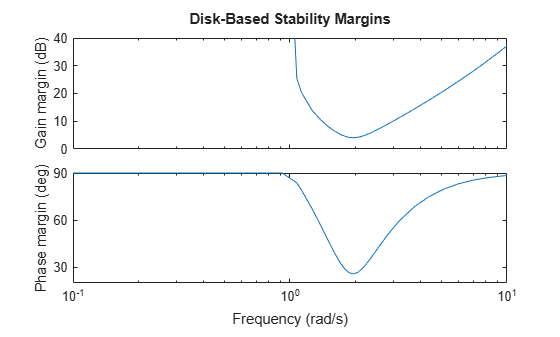The disk-based gain margin at each frequency is at least `±GM`, where `GM` is the value shown in the plot in dB. Similarly, the disk-based phase margin is `±PM` degrees. The disk-based margins returned by the `diskmargin` command are the smallest margins over frequency. (Right-click on the plot and select Characteristics > Minimum Disk Margin for a data tip containing information about these minimum margins.)

Compare the disk-based gain and phase margins of two open-loop responses on the same plot.

```L1 = tf(25,[1 10 10 10]); L2 = tf([1 100],[1 10 20 50]); diskmarginplot(L1,L2)```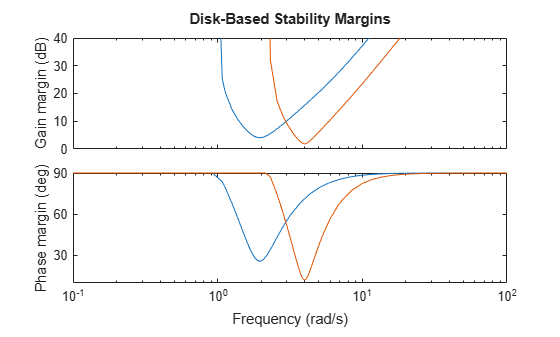You can customize the appearance of the plots using the `LineSpec` argument. Plot the margins again, using a blue dashed line for `L1` and red dots for `L2`.

`diskmarginplot(L1,'b--',L2,'r.')`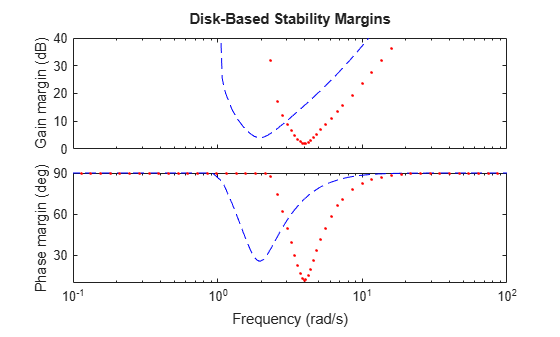The disk-based gain margins returned by `diskmargin` assume no phase variation, and the phase margins assume no gain variation. In practice, systems experience both gain and phase variation at the same time. Disk-margin analysis accounts for such simultaneous gain and phase variation.

Compute the disk-based gain and phase margins of a system. Then, use `diskmarginplot` to visualize the corresponding allowed ranges of simultaneous gain and phase variation.

```L = tf(25,[1 10 10 10]); DM = diskmargin(L); DGM = DM.GainMargin```
```DGM = 1×2 0.6273 1.5942 ```
`DPM = DM.PhaseMargin`
```DPM = 1×2 -25.8017 25.8017 ```
`diskmarginplot(DGM)`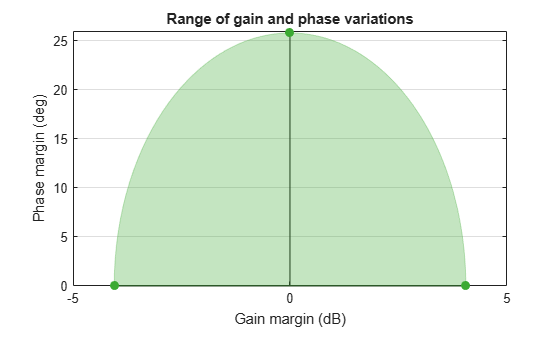The shaded region in the plot shows the range of simultaneous gain and phase variations that preserve the stability of the closed-loop system `feedback(L,1)`. When there is no phase variation, the system can tolerate the full gain-variation range `DGM`, from –4 dB to 4 dB. If the phase is allowed to vary by about ±20 degrees, the allowable gain-variation range drops to about –2.5 dB to 2 dB. At the full phase-variation range of ±26 degrees, the system can tolerate no gain variation.

To visualize multiple ranges on the same plot, combine them into a two-column vector. For instance, compute the disk margins of L with positive and negative skew and plot all three ranges of variation together.

```DMn = diskmargin(L,-2); DGMn = DMn.GainMargin; DMp = diskmargin(L,2); DGMp = DMp.GainMargin; DGMall = [DGMn;DGM;DGMp]; diskmarginplot(DGMall)```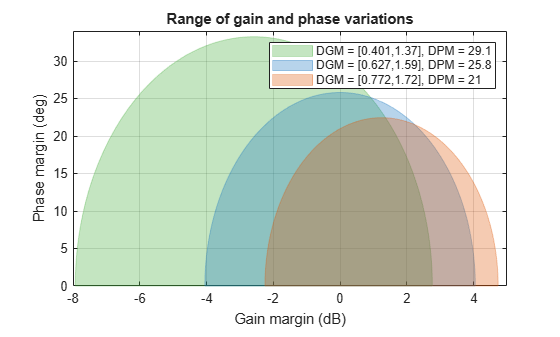This plot shows that the feedback loop can tolerate larger gain and phase variations when the gain decreases. In other words, the loop stability is more sensitive to gain increase. For more information about how varying skew affects disk-based gain and phase margin estimates, see Stability Analysis Using Disk Margins.

Disk-based gain-margin analysis models gain and phase variation as an uncertain factor F, multiplying an open-loop gain L. (For details of this model, see Stability Analysis Using Disk Margins.) The stable range of gain and phase variations returned by the `diskmargin` command is equivalent to a disk of F values for which the closed loop is stable. When you obtain the disk-based gain and phase margins of a system, you can use `diskmarginplot` to visualize the corresponding disk of F values.

```L = tf(25,[1 10 10 10]); DM = diskmargin(L); DGM = DM.GainMargin```
```DGM = 1×2 0.6273 1.5942 ```
`diskmarginplot(DGM,'disk')`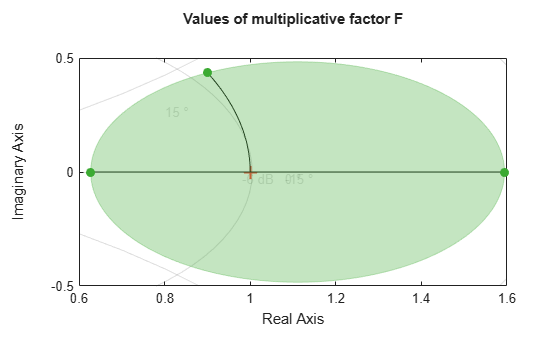The plot shows the values of F in the complex plane, where the x-axis is the real part and the y-axis is the imaginary part. The disk-based gain margin `DGM` uniquely determines this disk and the corresponding disk-based phase margin, `DPM`.

The uncertainty disk F is parameterized by two values: α, which sets the size of the disk, and σ, which biases the gain variation toward gain increase or decrease. This parameterization is given by:

$\mathit{F}=\frac{1+\alpha \left[\left(1-\sigma \right)/2\right]\delta }{1-\alpha \left[\left(1+\sigma \right)/2\right]\delta }$,

where δ is a normalized uncertainty. (For details, see Stability Analysis Using Disk Margins.) For a given range of gain variation, you can use `gm2dm` to convert the disk-based gain margin `DGM` to the α and σ values that describe the corresponding disk. `diskmarginplot` can plot the F disk for a given α,σ pair.

`DGM = [0.8,2]`
```DGM = 1×2 0.8000 2.0000 ```
```[alpha,sigma] = gm2dm(DGM); diskmarginplot(alpha,sigma,'disk')```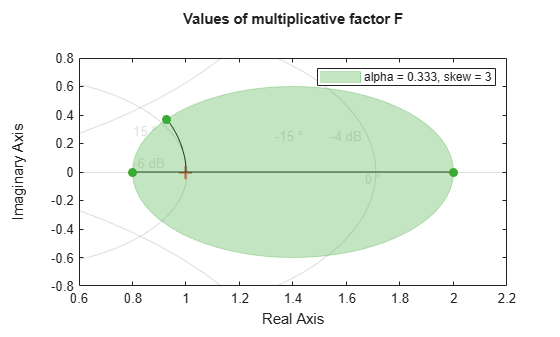In this case, σ is greater than zero because the disk-based gain range `DGM` = [0.8,2] includes more gain increase than decrease. σ = 0 represents a gain that can increase as much as it can decrease. σ < 0 represents a range with more decrease than increase. Plot the F disk for different values of σ to see how the gain range (the diameter of the disk) varies with σ.

```sigma = [-3,0,3]; diskmarginplot(alpha,sigma,'disk')```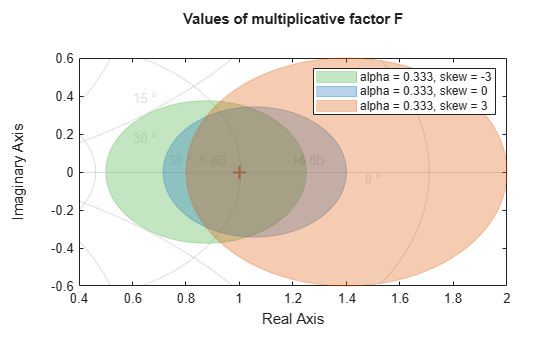For a fixed σ, α controls the size of the disk, and hence the amount of modeled uncertainty. Plot the disk for several values of α at σ = 0.

```sigma = 0; alpha = [0.1,0.333,0.5]; diskmarginplot(alpha,sigma,'disk')```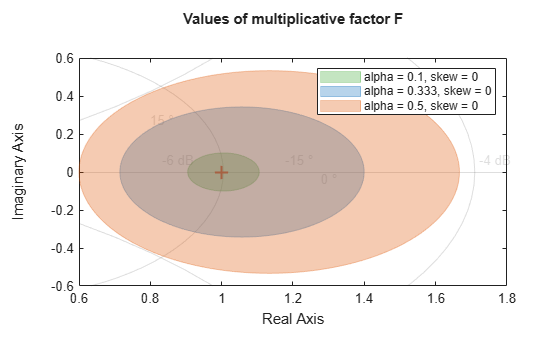As described in Stability Analysis Using Disk Margins, for a given σ, the largest uncertainty disk F for which the closed-loop system `feedback(L*F,1)` remains stable can be interpreted as an exclusion region that the Nyquist curve of L cannot enter. For any value of σ, the exclusion disk contains the critical point (–1,0) and is tangent to the Nyquist curve. The skew adjusts the size and position of the tangent disks. You can use `diskmarginplot` to visualize these exclusion disks and superimpose them on the Nyquist curve of L.

Compute disk-based gain margins for a system using three different skew values, one corresponding to more gain increase than decrease (σ > 0), one corresponding to more gain decrease than increase (σ < 0), and one balanced (σ = 0).

```L = tf(25,[1 10 10 10]); DMdec = diskmargin(L,-2); DGMdec = DMdec.GainMargin; DM = diskmargin(L,0); DGM = DM.GainMargin; DMinc = diskmargin(L,2); DGMinc= DMinc.GainMargin;```

To view the corresponding exclusion regions, plot the Nyquist plots of L and hold the figure. Then use `diskmarginplot` with the `'nyquist'` flag to add the exclusion regions to the plot.

```nyquist(L) hold on diskmarginplot([DGMdec;DGM;DGMinc],'nyquist') hold off```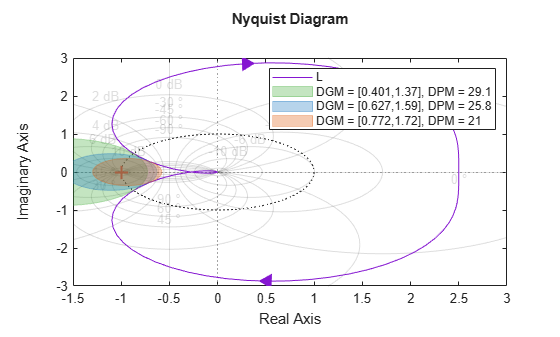As σ increases from –2 to 2, the disks move to the right, and each disk provides lower estimates of the classical gain and phase margins.

Plot the disk margins as a function of frequency of a system with the following open-loop response.

`L = tf(25,[1 10 10 10]);`

For the plot, specify the following attributes:

• Frequency units: Hz

• Gain margins on a log scale, in absolute units

• Grid on

```opts = diskmarginoptions; opts.FreqUnits = 'Hz'; opts.MagScale = 'log'; opts.MagUnits = 'abs'; opts.grid = 'on'; diskmarginplot(L,opts)```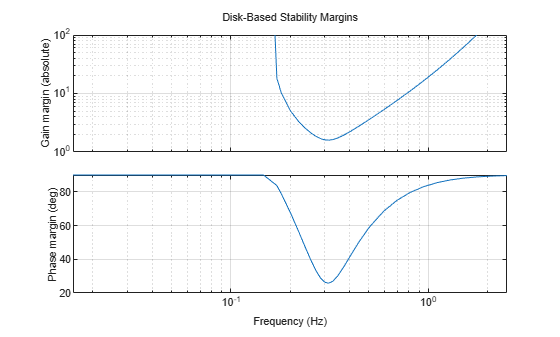## Input Arguments

collapse all

Open-loop response, specified as a dynamic system model. `L` can be SISO or MIMO, as long as it has the same number of inputs and outputs. `diskmarginplot` plots the disk-based gain and phase margins for the negative-feedback closed-loop system `feedback(L,eye(N))`.To plot the margins of the positive feedback system `feedback(L,eye(N),+1)`, use `diskmargin(-L)`.

If `L` is an uncertain state-space model (`uss` or `genss` with uncertain blocks), then `diskmarginplot` plots the margins of random samples of `L`. To visualize the worst-case stability margins of an uncertain system, use `wcdiskmarginplot`.

If `L` is a frequency-response data model (such as `frd`), then `diskmarginplot` plots the margins at each frequency represented in the model.

If `L` is a model array, then `diskmarginplot` plots the margins for all models in the array on the same axis and in the same line style.

Line style, marker, and color, specified as a string or vector of one, two, or three characters. The characters can appear in any order. You do not need to specify all three characteristics (line style, marker, and color). For example, if you omit the line style and specify the marker, then the plot shows only the marker and no line. For more information about configuring this argument, see the `LineSpec` input argument of the `plot` function.

Example: `'r--'` specifies a red dashed line

Example: `'*b'` specifies blue asterisk markers

Example: `'y'` specifies a yellow line

Skew of uncertainty region used to compute the stability margins, specified as a real scalar or vector (for `diskmarginplot(alpha,sigma)` plots only).

This parameter biases the uncertainty used to model gain and phase variations toward gain increase or gain decrease.

• The default `sigma` = 0 uses a balanced model of gain variation in a range `[gmin,gmax]`, with ```gmin = 1/gmax```.

• Positive `sigma` uses a model with more gain increase than decrease (`gmin > 1/gmax`).

• Negative `sigma` uses a model with more gain decrease than increase (`gmin < 1/gmax`).

For more detailed information about how the choice of `sigma` affects the margin computation, see Stability Analysis Using Disk Margins.

#### Skew in `diskmargin(L,sigma)` Syntax

When plotting the gain margins of a dynamic system versus frequency, use the default `sigma` = 0 to get unbiased estimates of gain and phase margins. For `sigma` = 0, the disk-based gain margin at each frequency is ±GM, where GM is the value shown in the plot in dB.

If you have used `diskmargin` to obtain disk-based margins with some particular `sigma`, you can use this syntax to see the frequency dependence of the margins at that `sigma` value. For `sigma` ≠ 0, the plotted value is ```GM = min(gmax,1/max(0,gmin))```. In other words, the plot shows the largest amount of gain change `[1/GM,GM]` that fits within the disk-based gain margin `[gmin,gmax]` of the system at the specified sigma.

For the syntax `diskmarginplot(L,sigma)`, the skew `sigma` must be a scalar.

#### Skew in `diskmargin(alpha,sigma)` Syntax

In the syntax `diskmargin(alpha,sigma)`, the function plots the uncertainty disk parameterized by the values `alpha` and `sigma` (see Stability Analysis Using Disk Margins). To convert between disk-based gain margins and `alpha`, `sigma` parameterization, use `dm2gm` and `gm2dm`. For this syntax, `diskmarginplot` enforces the relation `alpha*abs(1+sigma)` < 2.

For this syntax, the skew `sigma` can be a vector, allowing you to compare multiple disks on the same plot. If `alpha` is a scalar and `sigma` is a vector, then the plot shows the disks corresponding to the pairs `alpha,sigma_k` for each entry in `sigma`. If both `alpha` and `sigma` are vectors, then the plot shows the disks for the pairs `alpha1,sigma1;...;alphaN,sigmaN`.

Frequencies at which to plot stability margins, specified as the cell array `{wmin,wmax}` or as a vector of frequency values.

• If `w` is a cell array of the form `{wmin,wmax}`, then the plot shows the margins at frequencies between `wmin` and `wmax`.

• If `w` is a vector of frequencies, then the plot shows the margins at each specified frequency. For example, use `logspace` to generate a row vector with logarithmically spaced frequency values.

Specify frequencies in units of rad/`TimeUnit`, where `TimeUnit` is the `TimeUnit` property of `L`.

Plot options, specified as a `diskmarginplot` options set that you create with `diskmarginoptions`. Elements that you can customize include plot title, axes labels, and grids.

Disk-based gain margin, specified as a scalar, a two-element vector of the form `[gmin,gmax]`, or a two-column matrix of the form `[gmin1,gmax1; ...; gminN,gmaxN]`.

Use a two-element vector, `DGM` = `[gmin,gmax]` to plot the allowable range of simultaneous gain and phase variations corresponding to the disk-based gain margin `[gmin,gmax]`. You can obtain `[gmin,gmax]` in the `GainMargin` field of the output structures of the `diskmargin` command. You can also obtain `[gmin,gmax]` from classical gain and phase margins using `getDGM`.

Using a scalar `DGM` is equivalent to specifying the symmetric gain margin `[1/DGM,DGM]`.

To show the ranges of several disk-based gain margins on the same plot, use `DGM` = `[gmin1,gmax1;...;gminN,gmaxN]`. For symmetric gain margins of the form `[1/gmax,gmax]`, you can use a column vector of the form `[gmax1;gmax2;...;gmaxN]`.

Size of modeled gain and phase variation, specified as a scalar or vector. Disk-based gain-margin analysis models gain and phase variation as a multiplicative uncertainty F, which is a disk of values containing F = 1, corresponding to the nominal value of the system. The disk is parameterized by `alpha`, which sets the size of the disk, and `sigma`, which biases the gain variation toward gain increase or decrease. (For details of this model, see Stability Analysis Using Disk Margins.)

`diskmarginplot` lets you plot the disk F or the region of gain and phase variations represented by particular `alpha`,`sigma` pairs. For the syntax `diskmarginplot(alpha,sigma)`, the disk size `alpha` can be a vector. If `alpha` is a vector and `sigma` is a scalar, then the plot shows the regions corresponding to the pairs `alpha_k,sigma` for each entry in `alpha`. If both `alpha` and `sigma` are vectors, then the plot shows the regions for the pairs `alpha1,sigma1;...;alphaN,sigmaN`.

`diskmarginplot` enforces the relation `alpha*abs(1+sigma)` < 2. To convert between disk-based gain margins and `alpha`, `sigma` parameterization, use `dm2gm` and `gm2dm`.

Axes handle, specified as an `axes` object or a `UIaxes` object. Use this argument to specify axes when creating apps in Develop Apps Using App Designer.

 Seiler, Peter, Andrew Packard, and Pascal Gahinet. “An Introduction to Disk Margins [Lecture Notes].” IEEE Control Systems Magazine 40, no. 5 (October 2020): 78–95.